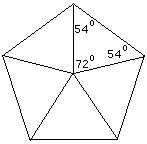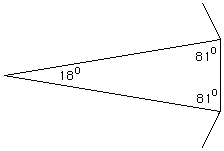```Date: Wed, 4 Dec 1996 21:43:06 -0600 (CST)

Subject: Geometry

Name:          Rick

Who is asking: Parent
Level:         Secondary
```
If you are given the measure of each interior angle (162 degrees) of a regular polygon. how many sides does the polygon have?
Hi Rick

If you look at a pentagon first where the interior angle is 108 deg then you see.The angle at the center is 180-108=72 deg and 360/72=5
Now in your problem where the interior angle is 162 degThe angle at the center is 180-162=18 deg and 360/18=20 so the polygon has 20 sides.
Penny

Go to Math Central

To return to the previous page use your browser's back button.